# Distributivity

(diff) ← Older revision | Latest revision (diff) | Newer revision → (diff)

distributivity law, distributive property, of one operation with respect to another

The property of a pair of binary algebraic operations (cf. Algebraic operation), expressed by one of the following identities: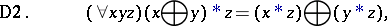whereare the symbols of the binary operations, and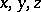are object variables. If in a settwo specific binary operations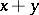,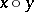are defined, i.e. two mappings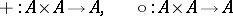are given, and the symbols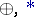are interpreted as the symbols of the respective operations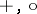in, one can speak of the truth or falsehood of each one of the formulas D1 and D2 in. If both these formulas are true in, the operationis called distributive with respect to the operation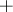in.

I.e., inthe operationis distributive with respect toif for allone hasand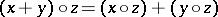.